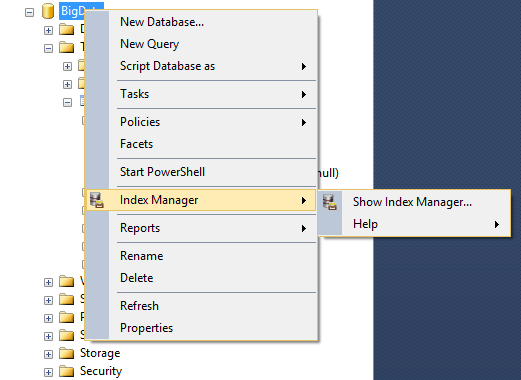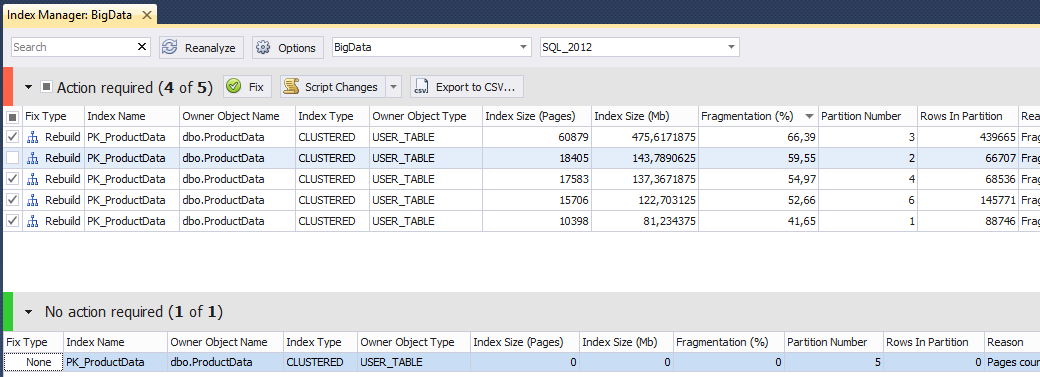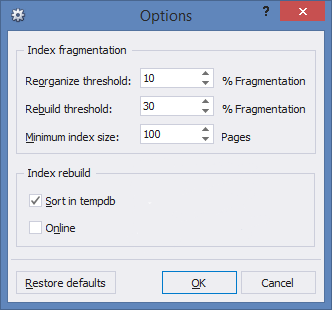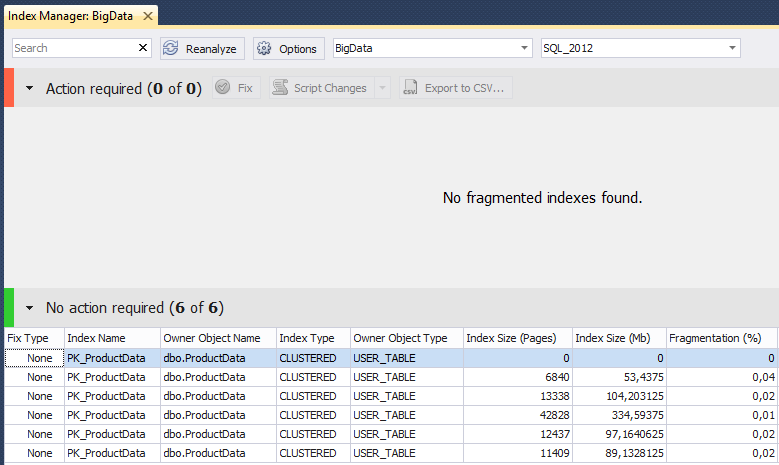# SQL SERVER – Fix SQL Server Index Fragmentation with dbForge Index Manager

You need to know SQL Server basics to keep database performance at the highest level. This knowledge will also help you to be prepared to any potential problems. When working with files, you may discover that there is not enough free space to store the required data in the file. By default, in such situations, SQL Server locks the file and extends it (it’s called – autogrow). Let us learn about SQL Server Index Fragmentation.

All autogrow events are stored in SQL Server log:

```SELECT
DatabaseName
, [FileName]
, CONVERT(VARCHAR(20), EndTime - StartTime, 114)
, StartTime
, EndTime
, FileType =
CASE EventClass
WHEN 92 THEN 'Data'
WHEN 93 THEN 'Log'
END
FROM (
SELECT pt = REVERSE(SUBSTRING(REVERSE([path]), CHARINDEX('\', REVERSE([path])), 260)) + N'log.trc'
FROM sys.traces
WHERE is_default = 1
) p
CROSS APPLY sys.fn_trace_gettable(pt, DEFAULT)
WHERE EventClass IN (92, 93)
ORDER BY StartTime DESC
```

Frequent call of autogrow files may significantly reduce productivity and may also lead to the fragmentation of files on the disk. The following settings (that differ from the recommended) may affect this call:

```IF OBJECT_ID('tempdb.dbo.#temp') IS NOT NULL
DROP TABLE #temp
GO
CREATE TABLE #temp (
db SYSNAME DEFAULT DB_NAME(),
flname SYSNAME,
size_after_growth DECIMAL(18,2),
size DECIMAL(18,2),
space_used DECIMAL(18,2),
growth INT,
is_percent_growth BIT,
PRIMARY KEY CLUSTERED (db, flname)
)
DECLARE @SQL NVARCHAR(MAX)
SELECT @SQL = STUFF((
SELECT '
USE [' + name + ']
INSERT INTO #temp (flname, size_after_growth, space_used, size, growth, is_percent_growth)
SELECT
name
, CASE WHEN is_percent_growth = 1 THEN size * (1 + (growth / 100.)) ELSE size + growth END * 8. / 1024
, space_used = FILEPROPERTY(name, ''SpaceUsed'') * 8. / 1024
, size = size * 8. / 1024
, CASE WHEN is_percent_growth = 1 THEN growth ELSE growth * 8. / 1024 END
, is_percent_growth
FROM sys.database_files'
FROM sys.databases
WHERE [state] = 0
FOR XML PATH(''), TYPE).value('.', 'NVARCHAR(MAX)'), 1, 2, '')
EXEC sys.sp_executesql @SQL
SELECT
db
, flname
, size_after_growth
, size
, space_used
, CAST(growth AS VARCHAR(10)) + CASE WHEN is_percent_growth = 1 THEN ' %' ELSE ' MB' END
FROM #temp
WHERE (is_percent_growth = 0 AND growth &amp;lt; 50)
OR (is_percent_growth = 1 AND growth &amp;lt; 5)
OR (size - space_used &amp;lt; 20)
```

If this query will return the 1 MB in the last column for some files, then think about the situation when we need to insert 100 MB of data. Each time SQL Server will block file and increase it by 1 MB, and then paste the data into it. I would advise you to keep enough space for log and database files.

There are also two types of files fragmentation:

Logical fragmentation (also called external fragmentation or extent fragmentation) — the logical order of the pages does not correspond their physical order. As a result, SQL Server increases the number of physical (random) reads from the hard drive, making the read-ahead mechanism less efficient. This directly affects to the query execution time, because random reading from the hard drive is far less efficient comparing to sequential reading.

Internal fragmentation — the data pages in the index contain free space. This lead to an increase in the number of logical reads during the query execution, because the index utilizes more data pages to store data.

To manage index fragmentation issues, SQL Server provides two statements: ALTER INDEX REBUILD / REORGANIZE.

The REBUILD operation creates a new structure for the index. The REORGANIZE operation is more lightweight. It runs through the leaf level of the index, and as it goes, it fixes physical ordering of pages and also compacts pages to apply any previously set fillfactor settings.

Do not ignore high index fragmentation level. You can get the information about the fragmentation by executing the following query:

```SELECT
SCHEMA_NAME(o.[schema_id]) AS [schema_name]
, o.name AS parent_name
, i.name
, s.avg_fragmentation_in_percent
, s.avg_page_space_used_in_percent
, i.type_desc
, s.page_count
, size = s.page_count * 8. / 1024
, p.partition_number
, p.[rows]
FROM sys.dm_db_index_physical_stats(DB_ID(), NULL, NULL, NULL, 'DETAILED') s
JOIN sys.partitions p ON s.[object_id] = p.[object_id] AND s.index_id = p.index_id
AND s.partition_number = p.partition_number
JOIN sys.indexes i ON i.[object_id] = s.[object_id] AND i.index_id = s.index_id
JOIN sys.objects o ON o.[object_id] = i.[object_id]
WHERE i.is_disabled = 0
AND i.is_hypothetical = 0
AND s.index_level = 0
AND s.page_count &amp;gt; 0
AND i.[type] &amp;gt; 0
AND s.avg_fragmentation_in_percent &amp;gt; 15
AND s.alloc_unit_type_desc = 'IN_ROW_DATA'
AND o.[type] IN ('U', 'V')
ORDER BY s. avg_fragmentation_in_percent DESC
```

Depending on the level of fragmentation, you can generate scripts to automatically rebuild or reorganize indexes:

```DECLARE @SQL NVARCHAR(MAX)
SELECT @SQL = (
SELECT '
ALTER INDEX [' + i.name + N'] ON [' + SCHEMA_NAME(o.[schema_id]) + '].[' + o.name + '] ' +
CASE WHEN s.avg_fragmentation_in_percent &amp;gt; 30
THEN 'REBUILD'
ELSE 'REORGANIZE'
END + ';
'
FROM (
SELECT
s.[object_id]
, s.index_id
, avg_fragmentation_in_percent = MAX(s.avg_fragmentation_in_percent)
FROM sys.dm_db_index_physical_stats(DB_ID(), NULL, NULL, NULL, NULL) s
WHERE s.page_count &amp;gt; 128 -- &amp;gt; 1 MB
AND s.index_id &amp;gt; 0 -- &amp;lt;&amp;gt; HEAP
AND s.avg_fragmentation_in_percent &amp;gt; 5
GROUP BY s.[object_id], s.index_id
) s
JOIN sys.indexes i ON s.[object_id] = i.[object_id] AND s.index_id = i.index_id
JOIN sys.objects o ON o.[object_id] = s.[object_id]
FOR XML PATH(''), TYPE).value('.', 'NVARCHAR(MAX)')
PRINT @SQL
EXEC sys.sp_executesql @SQL
```

However, it may be too tough task for the novice users. Moreover, I prefer simple solutions. Recently, Devart was announced a new add-in for SSMS – dbForge Index Manager for SQL Server. The new tool will be used to analyze and fix index fragmentation for databases.

Let’s create a test database and populate it with test data. For this, we can use dbForge Data Pump.

```USE [master]
GO
IF DB_ID('BigData') IS NOT NULL DROP DATABASE [BigData]
GO
CREATE DATABASE [BigData]
GO
USE [BigData]
GO
CREATE PARTITION FUNCTION [TypeID_PF](tinyint) AS RANGE LEFT FOR VALUES (0x14, 0x96, 0x97, 0xAE, 0xAF)
GO
CREATE PARTITION SCHEME [PD_PS] AS PARTITION [TypeID_PF] TO ([PRIMARY], [PRIMARY], [PRIMARY], [PRIMARY], [PRIMARY], [PRIMARY])
GO
CREATE TABLE [dbo].[ProductData] (
[ProductID] [int] NOT NULL,
[TypeID] [tinyint] NOT NULL,
[Specification] [nvarchar](MAX) NOT NULL,
CONSTRAINT [PK_ProductData] PRIMARY KEY CLUSTERED
(
[ProductID] ASC,
[TypeID] ASC
)WITH (PAD_INDEX = OFF, STATISTICS_NORECOMPUTE = OFF, IGNORE_DUP_KEY = OFF) ON [PD_PS]([TypeID])
) ON [PD_PS]([TypeID])
GO
```

Open the Index Manager:The Index Manager will search for fragmented indexes and offer options to fix them:Click Fix to remove index fragmentation. Alternatively, you can automatically generate a script:

```USE BigData
GO
ALTER INDEX [PK_ProductData] ON [dbo].[ProductData] REBUILD PARTITION = 1
WITH (SORT_IN_TEMPDB = ON)
GO
ALTER INDEX [PK_ProductData] ON [dbo].[ProductData] REBUILD PARTITION = 2
WITH (SORT_IN_TEMPDB = ON)
GO
ALTER INDEX [PK_ProductData] ON [dbo].[ProductData] REBUILD PARTITION = 3
WITH (SORT_IN_TEMPDB = ON)
GO
ALTER INDEX [PK_ProductData] ON [dbo].[ProductData] REBUILD PARTITION = 4
WITH (SORT_IN_TEMPDB = ON)
GO
ALTER INDEX [PK_ProductData] ON [dbo].[ProductData] REBUILD PARTITION = 6
WITH (SORT_IN_TEMPDB = ON)
```

You can change the settings, if necessary:Now we can use a free tool — dbForge Event Profiler for SQL Server (as we did in previous post), and see what queries does Index Manager send.

For this, we can have a look at the trace, and see that there is not too much queries:

```-- SQL Server version and edition
SELECT SERVERPROPERTY('ProductVersion'), SERVERPROPERTY('EngineEdition')
-- list of user databases
SELECT database_id, name
FROM [master].sys.databases
WHERE is_in_standby = 0
AND state_desc = 'ONLINE'
AND source_database_id IS NULL
```

А query that returns fragmented indexes is quite complex:

```DECLARE @db_id INT
SET @db_id = DB_ID()
SELECT
SCHEMA_NAME(o.[schema_id]) AS [schema_name]
, o.name AS parent_name
, i.name
, s.avg_fragmentation_in_percent
, i.type_desc
, o.[type] AS object_type
, s.page_count
, p.partition_number
, p.[rows]
, ISNULL(lob.is_lob_legacy, 0) AS is_lob_legacy
, ISNULL(lob.is_lob, 0) AS is_lob
, CASE WHEN ds.[type] = 'PS' THEN 1 ELSE 0 END AS is_partitioned
FROM sys.dm_db_index_physical_stats(@db_id, NULL, NULL, NULL, NULL) s
JOIN sys.partitions p ON s.[object_id] = p.[object_id] AND s.index_id = p.index_id AND s.partition_number = p.partition_number
JOIN sys.indexes i ON i.[object_id] = s.[object_id] AND i.index_id = s.index_id
LEFT JOIN (
SELECT
c.[object_id]
, index_id = ISNULL(i.index_id, 1)
, is_lob_legacy = MAX(CASE WHEN c.system_type_id IN (34, 35, 99) THEN 1 END)
, is_lob = MAX(CASE WHEN c.max_length = -1 THEN 1 END)
FROM sys.columns c
LEFT JOIN sys.index_columns i ON c.[object_id] = i.[object_id] AND c.column_id = i.column_id AND i.index_id &amp;gt; 0
WHERE c.system_type_id IN (34, 35, 99)
OR c.max_length = -1
GROUP BY c.[object_id], i.index_id
) lob ON lob.[object_id] = i.[object_id] AND lob.index_id = i.index_id
JOIN sys.objects o ON o.[object_id] = i.[object_id]
JOIN sys.data_spaces ds ON i.data_space_id = ds.data_space_id
WHERE i.[type] IN (1, 2)
AND i.is_disabled = 0
AND i.is_hypothetical = 0
AND s.index_level = 0
AND s.alloc_unit_type_desc = 'IN_ROW_DATA'
AND o.[type] IN ('U', 'V')
```

I will tell you what it does. We can get fragmented indexes with help of the DMV (dm_db_index_physical_stats.) We can do this for the entire database (how it was shown earlier) or for specified table.

```USE AdventureWorks2012
GO
SELECT *
FROM sys.dm_db_index_physical_stats(DB_ID(), OBJECT_ID('Person.Person'), NULL, NULL, NULL)
```

If the compatibility level for your database is set to 80 (SQL Server 2000), the following query fails:

I would recommend writing such queries as follows:

```USE AdventureWorks2012
GO
DECLARE @db_id INT, @obj_id INT
SELECT @db_id = DB_ID(), @obj_id = OBJECT_ID('Person.Person')
SELECT *
FROM sys.dm_db_index_physical_stats(@db_id, @obj_id, NULL, NULL, NULL)
```

Query result displays a lot of useful information:

As you can see, only IN_ROW_DATA is fragmented.

IN_ROW_DATA – pages that store data columns in fixed-length columns. Variable-length data (no more than 8060 bytes) does not fit the IN_ROW_DATA page is stored as ROW_OVERFLOW_DATA page that has a link to IN_ROW_DATA. The data, which are larger than 8060 bytes, such as varchar(max), varchar(max), XML, TEXT, IMAGE is stored on the LOB_DATA pages.

The more free space on the page, the more internal fragmentation at IN_ROW_DATA pages. If the fragmentation level is high, SQL Server has to read a huge number of pages. This can increase the number of logical reads and decrease performance.

Now back to our query. The part of the query that may seems unclear:

```SELECT
c.[object_id]
, index_id = ISNULL(i.index_id, 1)
, is_lob_legacy = MAX(CASE WHEN c.system_type_id IN (34, 35, 99) THEN 1 END)
, is_lob = MAX(CASE WHEN c.max_length = -1 THEN 1 END)
FROM sys.columns c
LEFT JOIN sys.index_columns i ON c.[object_id] = i.[object_id] AND c.column_id = i.column_id AND i.index_id &amp;gt; 0
WHERE c.system_type_id IN (34, 35, 99)
OR c.max_length = -1
GROUP BY c.[object_id], i.index_id
```

It returns information about which tables use LOB columns. Why it is so important? SQL Server 2005 does not support online index rebuild for indexes which contain LOB columns. If you do not specify the ONLINE option, the index is unavailable while rebuilding. Here is an example that demonstrates why the LOB check is important:

```IF OBJECT_ID('dbo.test1', 'U') IS NOT NULL DROP TABLE dbo.test1
GO
CREATE TABLE dbo.test1 (ID INT PRIMARY KEY, [Text] NVARCHAR(MAX))
GO
ALTER INDEX ALL ON dbo.test1 REBUILD WITH(ONLINE=ON)
GO
```

SQL Server 2012 does not have such limitations for all data types (except deprecated IMAGE, TEXT, NTEXT).

```IF OBJECT_ID('dbo.test1', 'U') IS NOT NULL DROP TABLE dbo.test1
GO
CREATE TABLE dbo.test1 (ID INT PRIMARY KEY, [Text] NVARCHAR(MAX))
GO
ALTER INDEX ALL ON dbo.test1 REBUILD WITH(ONLINE=ON)
GO
IF OBJECT_ID('dbo.test2', 'U') IS NOT NULL DROP TABLE dbo.test2
GO
CREATE TABLE dbo.test2 (ID INT PRIMARY KEY, [Text] TEXT)
GO
ALTER INDEX ALL ON dbo.test2 REBUILD WITH(ONLINE=ON)
GO
```

Do not use deprecated data types. You can check it with the following query:

```SELECT DISTINCT
OBJECT_SCHEMA_NAME(c.[object_id])
, OBJECT_NAME(c.[object_id])
FROM sys.columns c
WHERE c.system_type_id IN (34, 35, 99)
```

I did not discover any problems, while exploring dbForge Index Manager for SQL Server. The tool is stable and performs a great job for me.Reference: Pinal Dave (https://blog.sqlauthority.com)

#### Related Posts

•December 14, 2015 11:53 pm

Pinal hello; Which version of magnament studio, where is the index manager option?

•Pinal Dave
December 26, 2015 5:35 pm

Its from dbForge not part of SSMS

•nima
December 5, 2018 2:55 pm

I Suggest this update to used in Columnstore cases:

DECLARE @SQL NVARCHAR(MAX)
SELECT @SQL = (
SELECT ‘
ALTER INDEX [‘ + i.name + N’] ON [‘ + SCHEMA_NAME(o.[schema_id]) + ‘].[‘ + o.name + ‘] ‘ +
CASE WHEN i.name LIKE ‘%CIX%’
THEN ‘rebuild WITH (DATA_COMPRESSION = COLUMNSTORE) ‘
ELSE ‘rebuild’
END + ‘;

FROM (
SELECT
s.[object_id]
, s.index_id
, avg_fragmentation_in_percent = MAX(s.avg_fragmentation_in_percent)
FROM sys.dm_db_index_physical_stats(DB_ID(), NULL, NULL, NULL, NULL) s
WHERE s.page_count > 128 —
AND s.index_id != 0 —
AND s.avg_fragmentation_in_percent > 30
GROUP BY s.[object_id], s.index_id
) s
JOIN sys.indexes i ON s.[object_id] = i.[object_id] AND s.index_id = i.index_id
JOIN sys.objects o ON o.[object_id] = s.[object_id]
FOR XML PATH(”), TYPE).value(‘.’, ‘NVARCHAR(MAX)’)
PRINT @SQL